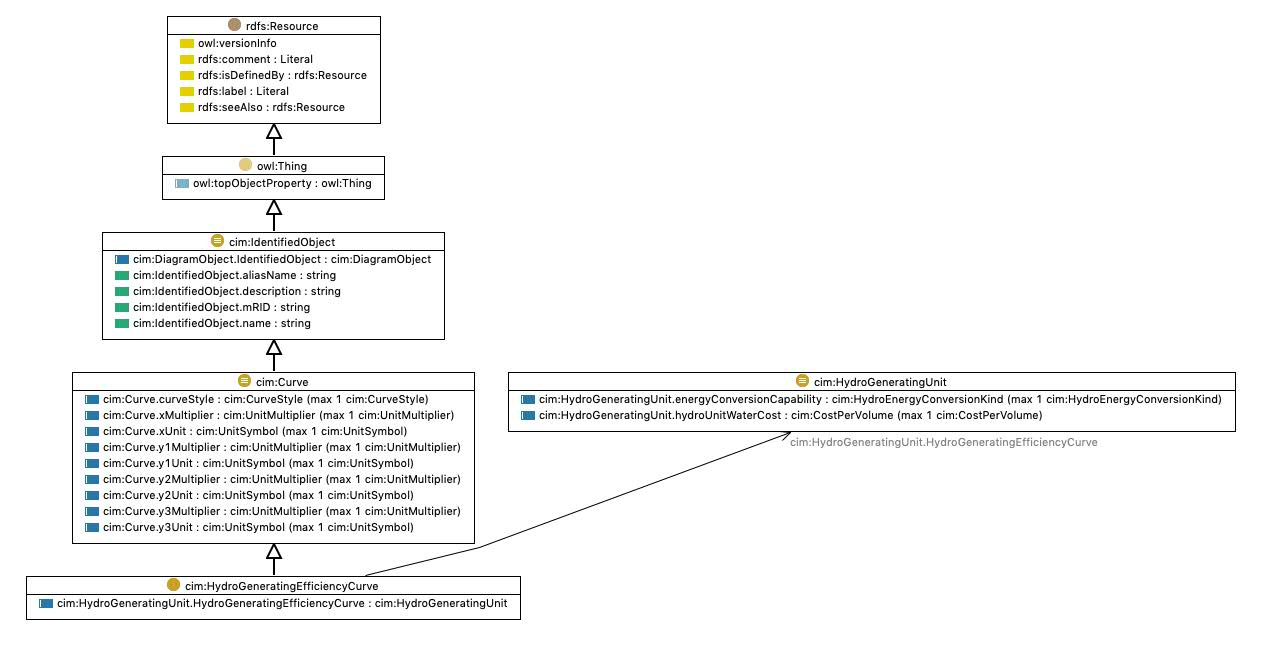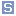## http://www.iec.ch/TC57/CIM#HydroGeneratingEfficiencyCurve Class cim:HydroGeneratingEfficiencyCurvetypeClass [owl:Class]
commentRelationship between unit efficiency in percent and unit output active power for a given net head in meters. The relationship between efficiency, discharge, head, and power output is expressed as follows: E =KP/HQ Where: (E=percentage) (P=active power) (H=height) (Q=volume/time unit) (K=constant) For example, a curve instance for a given net head could relate efficiency (Y-axis) versus active power output (X-axis) or versus discharge on the X-axis.
subClassOfcim:Curve
References

Generated with TopBraid Composer by TopQuadrant, Inc.